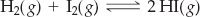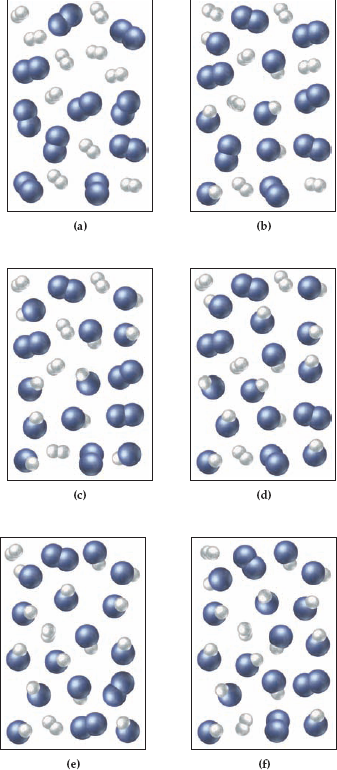×
Get Full Access to Introductory Chemistry - 5 Edition - Chapter 15 - Problem 107p
Get Full Access to Introductory Chemistry - 5 Edition - Chapter 15 - Problem 107p

×

# Solved: H2 and I2 are combined in a flask and allowed to react according to theISBN: 9780321910295 34

## Solution for problem 107P Chapter 15

Introductory Chemistry | 5th Edition

• Textbook Solutions
• 2901 Step-by-step solutions solved by professors and subject experts
• Get 24/7 help from StudySoup virtual teaching assistantsIntroductory Chemistry | 5th Edition

4 5 1 420 Reviews
25
2
Problem 107P

Problem 107P

H2 and I2 are combined in a flask and allowed to react according to the reaction:Examine the figures (sequential in time) and determine which figure represents the point where equilibrium is reached.Step-by-Step Solution:
Step 1 of 3

Solution: Here, we are going to determine the figure which represents the point of equilibrium for the given reaction.

Step1:

At the point of chemical equilibrium, the rates of forward and reverse reactions become equal and the concentrations of the reactants and the products remain constant.

The given reaction is:

H2(g) + I2(g) ⇄ 2HI(g)

In the series of diagram given, the number of reactants and products are:

1. H2 = 10, I2 = 10, HI = 0
2. H2 = 7, I2 = 7, HI = 6
3. H2 = 5, I2 = 5, HI = 10
4. H2 = 4, I2 = 4, HI = 12
5. H2 = 3, I2 = 3, HI = 14
6. H2 = 3, I2 = 3, HI = 14

As we have seen, on moving from figure (e) to (f), there is no change in the number of molecules, i.e., concentration of the reactants and the products.

Thus, figure (e) represents the point where equilibrium is reached.

---------------------

Step 2 of 3

Step 3 of 3

##### ISBN: 9780321910295

Unlock Textbook Solution